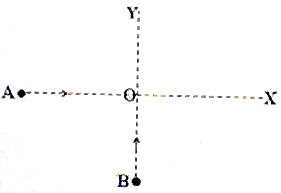## Pages

`“Life is like riding a bicycle.  To keep your balance you must keep moving.”–Albert Einstein`

## Sunday, December 14, 2008

### Fre-response Pracice Questions- AP Physics B&C- Work, Energy & Power

Two free-response questions involving work and energy are given below. The first question is meant for AP Physics B and the second question is meant for AP Physics C aspirants. If you are preparing for AP Physics C exam, you should be able to answer the questions meant for AP Physics B as well.(1) ABCDEF is a track which is straight and horizontal in the region BCDE but curved in the regions AB and EF. The track is smooth everywhere except over a length 1.5 m in the horizontal region CD where the coefficient of kinetic friction is 0.2. A block of mass m1 = 2 kg is released from rest from position A which is at a height of 3.2 m. It slides down and collides with another stationary block of mass m2 = 3 kg placed near position C (fig.). The velocity of the 3 kg mass m2 immediately after the collision is 4 ms–1. Now answer the following:

(a) Determine the velocity of the 2 kg mass m1 immediately after the collision.

(b) State whether this collision is elastic or inelastic. Justify your answer.

(c) Calculate the kinetic energy lost by the 3 kg mass during its forward trip along the rough track CD.

(d) Determine the maximum height ‘x up to which the 3 kg mass will rise along the smooth track EF.

(e) If the 2 kg mass m1 is removed (without disturbing any other thing) immediately after the collision, determine the distance from C where the 3 kg mass will finally come to rest.

The above question carries 15 points and you have about 17 minutes for answering it.

(2)
An object A of mass 5 kg moving along the positive x-direction has displacement x given by x = 0.2 t + 0.18 t2. The force acting on the object ceases after 5 seconds and the object moves with uniform velocity. Another object B of mass 10 kg moving in the positive y-direction has kinetic energy equal to the potential energy of a spring of force constant 3000 Nm–1 compressed through 0.1 m from its natural length. The objects A and B moving with their respective constant velocities collide at the origin O and stick together.(a) Determine the momentum and kinetic energy of object A just before collision.

(b) Determine the momentum and kinetic energy of object B just before collision

(c) Calculate the velocity of the combined mass (after A and B stick together).

(d) Calculate the change in kinetic energy of the system because of the collision.